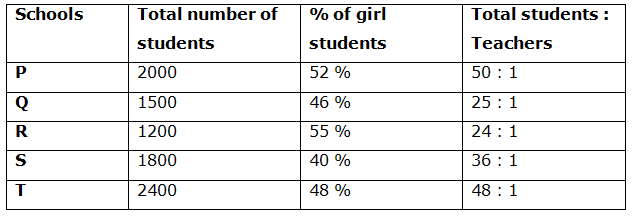# SBI Clerk Prelims 2020 Quantitative Aptitude Questions (Day-07)

Dear Aspirants, Our IBPS Guide team is providing new series of Quantitative Aptitude Questions for SBI Clerk Prelims 2020 so the aspirants can practice it on a daily basis. These questions are framed by our skilled experts after understanding your needs thoroughly. Aspirants can practice these new series questions daily to familiarize with the exact exam pattern and make your preparation effective.

[WpProQuiz 7627]

Ensure Your Ability Before the Exam – Take SBI Clerk 2020 Prelims Free Mock Test

Simplification

Directions (Q. 1 – 5): What value should come in the place of question mark in the given questions?

1) √625 × √6241 – 768 × 3 + √15129 + 106 × 2 = ?

a) 10

b) 16

c) 9

d) 6

e) None of these

2) 37.5 % of 80 % of 730 + 160 % of 250 – ? = 120 % of 400

a) 129

b) 139

c) 149

d) 159

e) None of these

3) 3 (3/4) + 4(2/5) – 3(1/8) + 6(2/5) = ?

a) 11(17/40)

b) 9(17/40)

c) 10(17/40)

d) 13(17/40)

e) None of these

4) √841 × 3 + 120 % of 450 – 180 % of 780 + 60 % of 1800 = ?

a) 303

b) 310

c) 308

d) 290

e) None of these

5) ? – (5/9) of 8379 – 243 ÷ 3 + 162 = 24

a) 4504

b) 3856

c) 5278

d) 2912

e) None of these

Data Interpretation

Directions (Q. 6 – 10): Study the following information carefully and answer the given questions.

Following table shows the total number of students and the percentage of girl students among them and the ratio of total number of students and teachers in 5 different schools.6) Total number of boy students in school Q is what percentage of total number of boys in school S?

a) 60 %

b) 75 %

c) 90 %

d) 80 %

e) None of these

7) Find the ratio between the total number of girl students in School P to that of School R?

a) 15: 8

b) 21: 13

c) 34: 15

d) 52: 33

e) None of these

8) Find the difference between the total number of teachers in school Q to that of school T?

a) 15

b) 25

c) 10

d) 20

e) None of these

9) Find the total number of teachers in school P, R and S together?

a) 140

b) 120

c) 105

d) 130

e) None of these

10) Total number of students in school P is approximately what percentage more/less than the total number of students in school R?

a) 40 % less

b) 55 % less

c) 95 % more

d) 70 % more

e) 80 % more

Directions (1-5) :

√625 × √6241 – 768 × 3 + √15129 + 106 × 2 = ?

25 * 79 – 2304 + 123 + 212 = ?

6=?

37.5 % of 80 % of 730 + 160 % of 250 – ? = 120 % of 400

219 + 400 – ? = 480

139 = ?

3 (3/4) + 4(2/5) – 3(1/8) + 6(2/5) = ?

(3 + 4 – 3 + 6) (3/4 + 2/5 – 1/8 + 2/5) = ?

10(57/40) = ?

11(17/40) = ?

√841 × 3 + 120 % of 450 – 180 % of 780 + 60 % of 1800 = ?

29 * 3 + 120/100 * 450 – 180/100 * 780 + 60/100 * 1800 =?

87 + 540 – 1404 + 1080 = ?

303 = ?

? – (5/9) of 8379 – 243 ÷ 3 + 162 = 24

? – (5/9)*8379 – (243/3) + 256 = 24

? – 4655 – 81 + 256 = 24

? = 24 + 4655 + 81 – 256 = 4504

Directions (6-10) :

Total number of boy students in school Q

= > 1500*(54/100) = 810

Total number of boys in school S

= > 1800*(60/100) = 1080

Required % = (810/1080)*100 = 75 %

The total number of girl students in School P

= > 2000*(52/100) = 1040

The total number of girl students in School R

= > 1200*(55/100) = 660

Required ratio = 1040 : 660 = 52 : 33

The total number of teachers in school Q

= > (1500/25) = 60

The total number of teachers in school T

= > (2400/48) = 50

Required difference = 60 – 50 = 10

The total number of teachers in school P, R and S together

= > (2000/50) + (1200/24) + (1800/36)

= > 40 + 50 + 50 = 140

Total number of students in school P = 2000

Total number of students in school R = 1200

Required % = [(2000 – 1200)/1200]*100 = 66.67 % = 70 % more

 Check Here to View SBI Clerk Prelims 2020 Quantitative Aptitude Questions Day 06 Day 05 Day  04 Click Here for SBI Clerk 2020 – Detailed Exam Notification